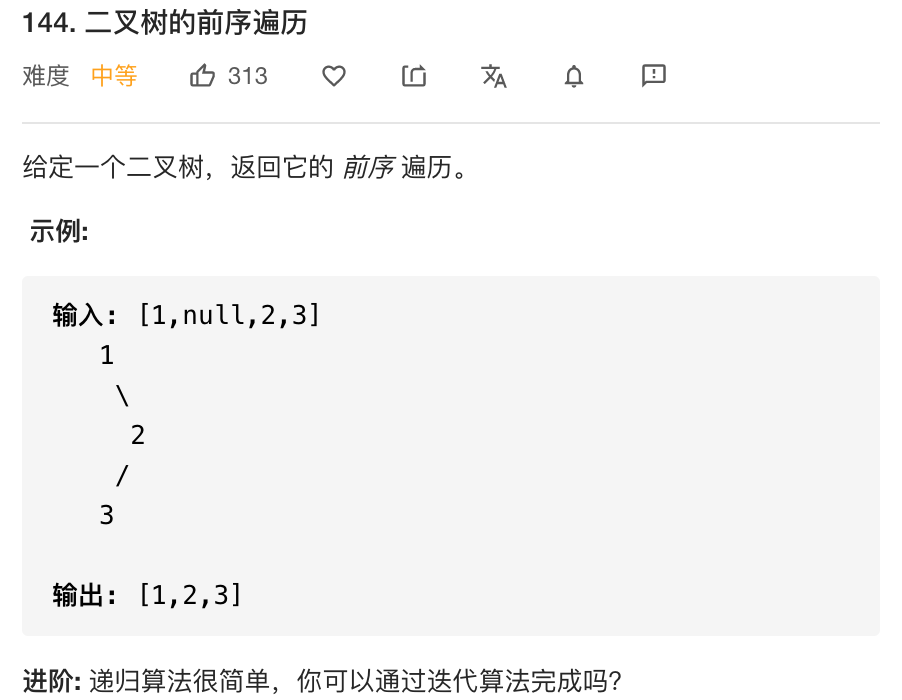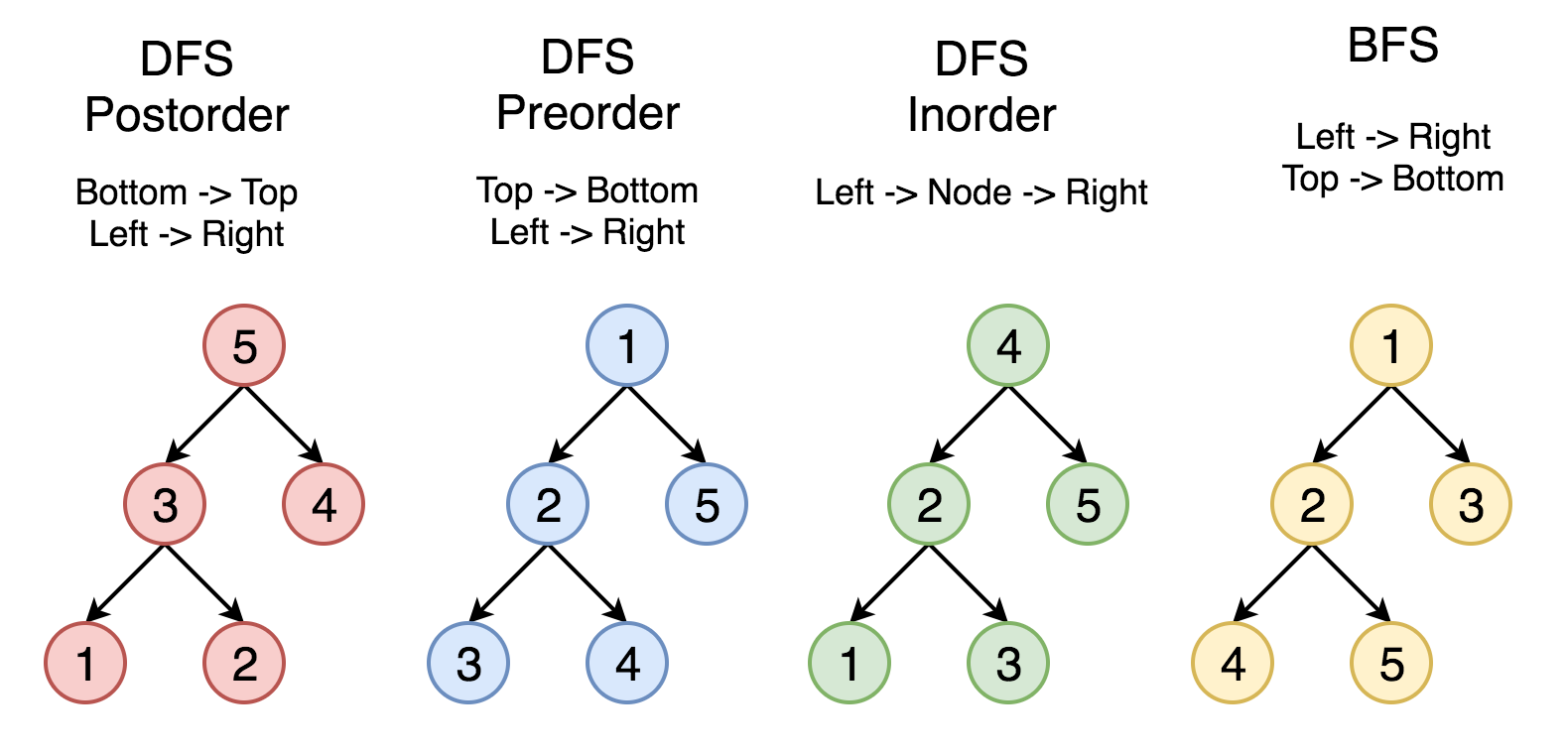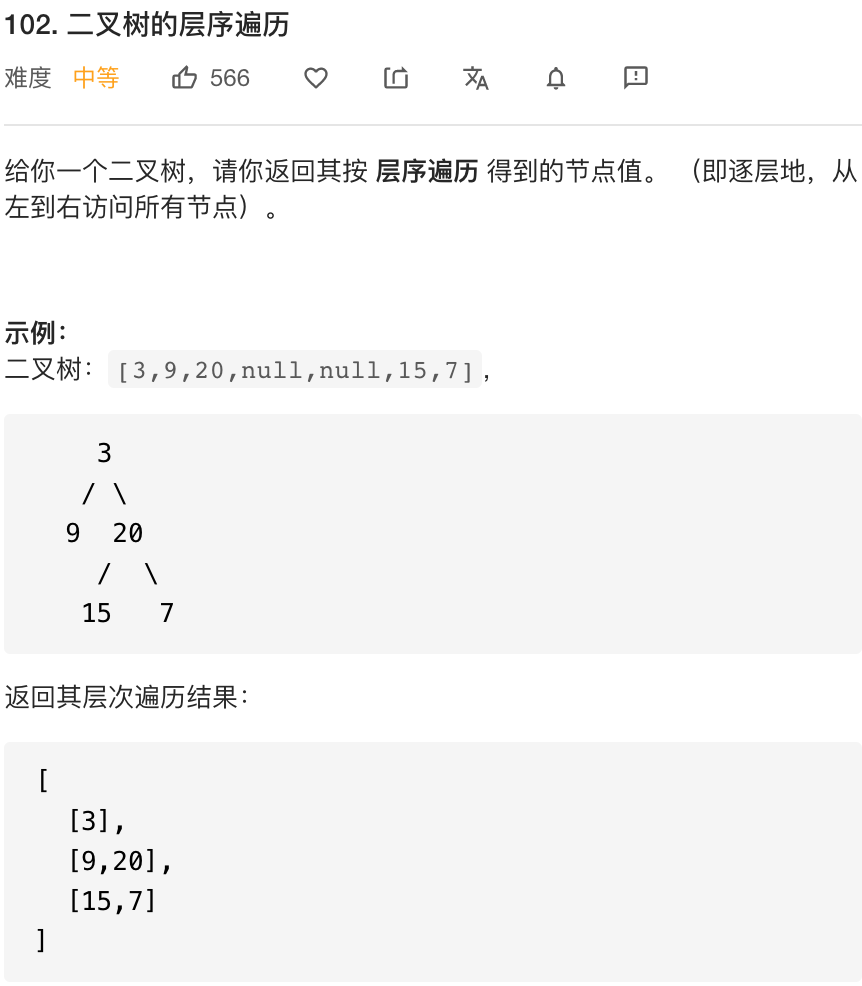# 二叉树遍历系列总结+递归/迭代的统一写法

## 一、题目

144. 二叉树的前序遍历
94 . 二叉树的中序遍历
145. 二叉树的后序遍历## 二、分析：DFS/BFS 与树的遍历

• 有两种通用的遍历树的策略：

• 深度优先搜索（DFS）

在这个策略中，我们采用深度作为优先级，以便从跟开始一直到达某个确定的叶子，
然后再返回根到达另一个分支。

深度优先搜索策略又可以根据根节点、左孩子和右孩子的相对顺序
被细分为前序遍历，中序遍历和后序遍历

• 宽度优先搜索（BFS）

我们按照高度顺序一层一层的访问整棵树，高层次的节点将会比低层次的节点先被访问到。
对应数的层次(层序遍历)

• *****五星重点

• 前中后序遍历的递归和迭代写法 本质上对应DFS的递归写法和显示调用栈的写法
• 递归解决方案的优点是它更容易实现。 但是，存在一个很大的缺点：如果递归的深度太高，你将遭受堆栈溢出。 在这种情况下，推荐使用显式栈实现 DFS(有时也可以用BFS)。
• 显式栈实现 DFS逻辑与递归解决方案完全相同。 但我们使用 while 循环和栈来模拟递归期间的系统调用栈
• DFS的两种方式的解题模板参考：深度优先搜索DFS_图文详解_Java代码_leetcode刷题模板
• DFS模板I—递归写法
/*
* Return true if there is a path from cur to target.
*/
boolean DFS(Node cur, Node target, Set<Node> visited) {
return true if cur is target;
for (next : each neighbor of cur) {
if (next is not in visited) {
return true if DFS(next, target, visited) == true;
}
}
return false;
}

• DFS模板II—显示调用栈写法
 /*
* Return true if there is a path from cur to target.
*/
boolean DFS(int root, int target) {
Set<Node> visited;
Stack<Node> s; //额外注意：BFS 这里使用的是queue队列。
while (s is not empty) {
Node cur = the top element in s;
return true if cur is target;
for (Node next : the neighbors of cur) {
if (next is not in visited) {
}
}
remove cur from s;
}
return false;
}## 三、递归的写法

• 1.前序遍历
class Solution {
public List<Integer> preorderTraversal(TreeNode root) {
//创建list
List<Integer> res = new ArrayList();

//递归调用
dfs(root,res);

//返回结果
return res;
}

private void dfs(TreeNode root, List<Integer> list){

if(root != null ){
//1.先添加root值

//2.递归左子树
dfs(root.left,list);

//3.递归右子树
dfs(root.right,list);
}

/* 【另一种写法】

//递归终止条件， base case
if(root == null) return;

//先遍历根节点，  直接添加节点值

//递归左子树
//if(root.left != null)
recur(root.left,list);

//递归右子树
//if(root.right != null)
recur(root.right,list);

*/
}
}

• 2.中序遍历
class Solution {
public List<Integer> inorderTraversal(TreeNode root) {
//创建list
List<Integer> res = new ArrayList();

//递归调用
dfs(root,res);

//返回结果
return res;
}

private void dfs(TreeNode root, List<Integer> list){

if(root != null ){

//1.递归左子树
dfs(root.left,list);

//2.添加root值

//3.递归右子树
dfs(root.right,list);
}

/* 【另一种写法】

//递归终止条件， base case
if(root == null) return;

//递归左子树
//if(root.left != null)
recur(root.left,list);

//遍历根节点，  直接添加节点值

//递归右子树
//if(root.right != null)
recur(root.right,list);

*/
}
}

• 3.后序遍历
class Solution {
public List<Integer> postorderTraversal(TreeNode root) {
//创建list
List<Integer> res = new ArrayList();

//递归调用
dfs(root,res);

//返回结果
return res;
}

private void dfs(TreeNode root, List<Integer> list){

if(root != null ){

//1.递归左子树
dfs(root.left,list);

//2.递归右子树
dfs(root.right,list);

//3.添加root值
}

/* 【另一种写法】

//递归终止条件， base case
if(root == null) return;

//递归左子树
//if(root.left != null)
recur(root.left,list);

//递归右子树
//if(root.right != null)
recur(root.right,list);

//遍历根节点，  直接添加节点值
*/
}
}


## 四、迭代写法

### 1. 不统一版本的迭代写法

• 前序遍历 标准的前序遍历的dfs显示调用栈的写法。(虽然这种写法很常见，但是无法中后序统一起来)

class Solution {
public List<Integer> preorderTraversal(TreeNode root) {
//递归写法  DFS + stack   和 BFS + queue的写法 很像
// java 中的 stack 栈 已经被LinkedList 取代了。
if(root == null) return res;//leetcode这里必须返回空集合，不能返回null

//dfs
while(!stack.isEmpty()){
//创建当前节点
TreeNode curr = stack.pollLast();// poll删除头部元素
//res 添加节点值
//stack添加curr的临近节点(左右子树)
//添加右子树 注意是右子树， 先进后出
//添加左子树节点
}

//返回结果
return  res;
}
}

• 中序遍历：中序遍历需要先让指针找到每颗子树的最最下角。

class Solution {
public List<Integer> preorderTraversal(TreeNode root) {

TreeNode curr = root;

while( curr != null || !stack.isEmpty()){

//先判断root.left 是否为空
if(curr != null){

//不为空 stack 添加节点

//curr指向左节点
curr = curr.left;
}else{
//如果curr.left为空 就添加curr到res
curr = stack.pollLast();

//curr 指向右节点
curr = curr.right;
}
}
return res;

}
}


class Solution {
public List<Integer> preorderTraversal(TreeNode root) {
//递归写法  DFS + stack   和 BFS + queue的写法 很像
// java 中的 stack 栈 已经被LinkedList 取代了。
if(root == null) return res;//leetcode这里必须返回空集合，不能返回null

//dfs
while(!stack.isEmpty()){
//创建当前节点
TreeNode curr = stack.pollLast();// poll删除头部元素
//res 添加节点值
//stack添加curr的临近节点(左右子树)
//添加右子树 注意是右子树， 先进后出
//添加左子树节点
}
//返回结果
return  res;
}
}


### 2. 统一版本的迭代写法

• 统一版本的迭代：其本质是用显示栈去模拟递归， 这里的模拟是真正做到了完全模拟递归，递归是怎么递归的，栈就是怎么模拟的。强烈建议用debug走一遍， 不要觉得看完前序遍历后，中序后序遍历只是调换一下顺序，正真的运行流程可能比你想象的复杂。
• 写法来源大佬PualKing在LeetCode的题解：完全模仿递归，不变一行。秒杀全场，一劳永逸
• 前序遍历
class Solution {
public List<Integer> preorderTraversal(TreeNode root) {
//创建res  和 stack

//异常处理
if(root == null) return  res;

//stack 初始化 根节点先入栈

while(!stack.isEmpty()){

//创建当前节点 stack弹出节点并判断是否访问过
TreeNode curr = stack.pollLast();

//非空说明没有访问过，然后右节点入栈，左节点入栈，最后根节点入栈，最后压入一个空节点(重要)
if( curr !=null){

}else{
//如果弹出的节点为空节点，表明当前栈顶节点已经访问过
}
}
//返回结果
return res;
}
}

• 中序遍历
class Solution {
public static List<Integer> inorderTraversal(TreeNode root) {

//创建res 和stack

//特例处理
if(root == null) return res;

//初始化 stack root 节点入栈

//dfs遍历
while(!stack.isEmpty()){

//创建当前节点  stack出栈
TreeNode curr = stack.pollLast();

//判断curr 是否为空，不为空就没有被访问过
//先添加右节点，然后添加curr节点， 再添加一个空节点，最后添加左节点
if(curr != null) {

}else{
//curr == null 说明已经被访问过
}
}
//返回结果
return res;
}
}

• 后序遍历
class Solution {
public static List<Integer> inorderTraversal(TreeNode root) {

//创建res 和stack

//特例处理
if(root == null) return res;

//初始化 stack root 节点入栈

//dfs遍历
while(!stack.isEmpty()){

//创建当前节点  stack出栈
TreeNode curr = stack.pollLast();

//判断curr 是否为空，不为空就没有被访问过
//添加curr节点，然后添加一个空节点，再添加右节点，最后添加左节点
if(curr != null) {

}else{
//curr == null 说明已经被访问过
}
}
//返回结果
return res;
}
}


## 五、复杂度分析

• 递归算法复杂度
时间复杂度：O(n)。递归函数 T(n) = 2 *T(n/2)+1。
空间复杂度：最坏情况下需要空间O(n)，平均情况为O(logn)。

• 迭代算法复杂度
时间复杂度：访问每个节点恰好一次，时间复杂度为 O(N) ，其中 NN 是节点的个数，也就是树的大小。
空间复杂度：取决于树的结构，最坏情况存储整棵树，因此空间复杂度是 O(N)。

• 链接：https://leetcode-cn.com/problems/binary-tree-postorder-traversal/solution/er-cha-shu-de-hou-xu-bian-li-by-leetcode/

## 六、层序遍历

• 层序遍历 直接套用BFS模版
• 题目：102.二叉树的层序遍历• 层序遍历
public class Solution {
//层序遍历  BFS + queue
public List<List<Integer>> levelOrder(TreeNode root) {

//特例处理
if(root == null) return res;

//初始化queue

while(!queue.isEmpty()) {
//这里需要额外记录下queue 的大小，主要为了处理完一层的节点后，方便返回一个List<Integer>
int n = queue.size();

//将当前队列的中的所有节点 向四周扩散
for(int i = 0 ; i < n; i++){
TreeNode curr = queue.pollFirst();

}
}

return res;

}
}

• 复杂度分析
记树上所有节点的个数为 nn。
时间复杂度O(n)：每个点进队出队各一次，故渐进时间复杂度为 O(n)。
空间复杂度O(n)：队列中元素的个数不超过 n 个，故渐进空间复杂度为 O(n)。
09-19157
06-25340208-22577
08-10142
07-12414
07-2222
10-241193
10-1153
07-2153
02-1278